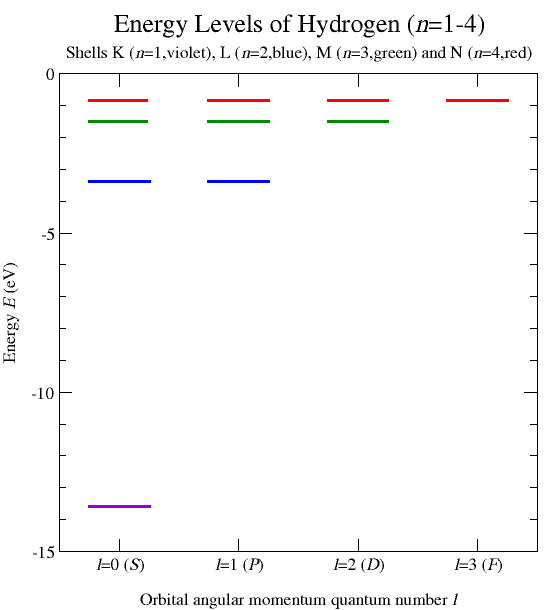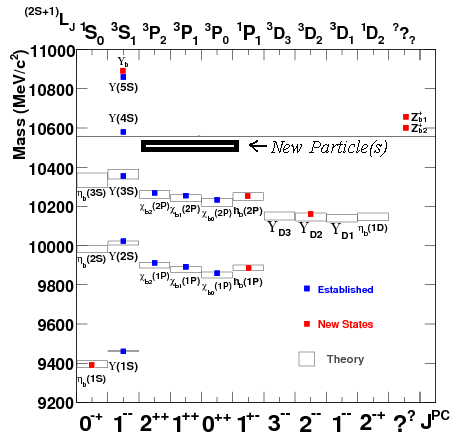## Thursday, December 29, 2011

### LHC: is $$\chi_b(3P)$$ a new particle?

Sometimes, quantitative progress isn't real progressA week ago, many media outlets including BBC and Fox News announced that the LHC at CERN has discovered its first new particle. It's called $$\chi_b(3P)$$; pronounce it "chi bee three pea". But is it fair to call it a new particle? And how excited should we be?

The preprint by the ATLAS collaboration released on December 22nd is called
Observation of a new $$\chi_b$$ state in radiative transitions to $$\Upsilon(1S)$$ and $$\Upsilon(2S)$$ at ATLAS (arXiv)
The "palm tree letter" above is known as "Upsilon" and if it sounds Greek to you, then it sounds exactly as it should.

Congratulations to ATLAS and especially the people most closely connected with the discovery.

The new particle is actually a level of a bottomonium, which is a bound state containing a bottom quark and the bottom antiquark, $$b\bar b$$, which is a subset of objects known as quarkonium. In a similar way, a charmonium is a $$c\bar c$$ bound state of the charm quark and the charm antiquark (the most famous level of this object is the $$J/\psi$$ meson). The term "quarkonium" isn't being used for the lighter quarks i.e. $$u,d,s$$ because these lighter quarks get mixed and the bound state doesn't have a pure enough "flavor". Despite the catchy name, a toponium $$t\bar t$$ doesn't exist because the top quark decays "before" it may create a bound state.

Bound states of quarks and antiquarks of different flavors (especially $$b\bar c$$ or $$c\bar b$$) also exist but it's unlikely for them to be produced because such a production violates two of the "charm", "bottom", or "top" quantum numbers.

At any rate, there's quite some potential for bound states. In the 1960s, people would know lots of related objects that were (and are) similar to those we call the "quarkonium" today: they interact by the strong nuclear interactions. Before the quarks/partons were discovered, all these particles were considered to be "kind of elementary" and a whole zoological garden of these creatures was waiting for some explanation.

Anthropic would-be physicists could have said that for our world to thrive and develop intelligent life, we may need a particular zoological garden of these beasts but their properties are otherwise random. Fortunately, physicists would find it disgusting to switch to this defeatist anthropic attitude in the 1960s. Instead of establishing an anthropic religion, they tried to find an explanation. As a result, they finally discovered both QCD and string theory. These two discoveries wouldn't be made if people failed to believe that there had to be an explanation of the seemingly contrived patterns in the jungle of the strongly interacting bound states.

But let's return to the end of 2011. As Matt Strassler pointed out, the term "new particle" may lead many readers to overestimate the importance of the discovery. The Wikipedia page I mentioned contains about twenty well-known levels of the bottomonium bound states. And a triplet of new ones was just found. Not an excessively big deal.

Each such level would be viewed as a new discovery before quarks were known and QCD was kind of understood. But the situation has been different for more than 35 years. We know QCD so we know that the bottomonium is analogous to the hydrogen atom. Here there are a few levels of this simplest atom:Via Matt's blog

As one may show by arguments based on the $$SO(4)$$ symmetry or by other methods, the $$1/r$$ interaction energy between the nucleus and the electron means that there are many levels given by the principal quantum number $$n=1,2,3,\dots$$ whose energy is, if we neglect relativistic and loop effects, $$-13.6\,\,{\rm eV}/n^2$$. For each $$n$$, the quantum number $$l$$ quantifying the orbital angular momentum can be anything between $$0$$ and $$n-1$$ while for each $$(n,l)$$, the $$z$$-component of the orbital angular momentum $$m=l_z$$ may be any integer between $$-l$$ and $$+l$$.

The potential energy between the bottom quark and its particle isn't given simply by $$1/r$$ so the problem is harder but the qualitative character of this bound state is similar: there are many discrete levels with various angular momenta and various energies. So roughly speaking, it's just like the hydrogen atom even though all the energies are different:Via Matt's blog

When you know that there are quarks that "orbit" each other and that may have wave functions corresponding to discrete energy eigenstates, you won't be shocked to find another one – one that may be a bit harder to be found than the previous ones but one that isn't "qualitatively different", anyway. Of course, one may invent "stories" and "contrived features" in which the newly found level is "the king", unprecedented, and better or harder or more stunning than the previous levels. Some of them explain why the level wasn't found before 2011. However, rationally speaking, all of this is just a hype. The discovery is intrinsically modest.

You could be satisfied with the discoveries of new bound states of well-known quarks – more precisely, new levels of well-known bound states of well-known quarks – but your intuition may be pretty good if you're not that excited. When people discovered the levels of the hydrogen atom, they were not distributing Nobel prizes for observing the $$n=7$$ and then $$n=8$$, $$n=9$$, and other levels of the hydrogen atom. They're just there. Everyone can see it.

A few decades after the best years of atomic physics, people focused on nuclear physics. This is the discipline investigating the processes inside the nuclei which are 10,000 times smaller than the atoms and where the typical energies are approximately 1,000,000 times larger – they are comparable to $$1\,\,{\rm MeV}$$ rather than the atomic chemical scale of $$1\,\,{\rm eV}$$. Nuclear physics depends on a stronger force than electromagnetism, namely the strong nuclear force, which is governed by nonlinear QCD rather than Maxwell's equations that obey the superposition principle, and all these differences make the calculations harder. But at the end, nuclear physics is very analogous to atomic physics, at least at the theoretical level. Even at the practical level, they're analogous: just the nuclear bombs that come out of the research are a million times stronger or more efficient than the "atomic physics" bombs (by which I really mean conventional explosives based on chemistry).

Quite generally, the incremental progress often becomes boring. When the levels of the hydrogen atom were found, a person with a very weak imagination could have thought that the future of physics would be about finding the $$n=10$$ level of the atom, then $$n=11$$, and so on, and he could predict that the physicists in 2011 would be thrilled by studying the $$n=754$$ level of the hydrogen atom. Instead, we are consumed by very different things. Increasing the value of the principal quantum number $$n$$ wasn't the only way to make progress. It wasn't the only way to extend or generalize the previous advances. Not at all.

People could have studied larger atoms and molecules and heavier counterparts of the electron and analogous forces that act inside the nuclei, and so on. But in some sense, even the very process of switching to ever heavier elementary particles may become boring and "conceptually old-fashioned". We, the curious physicists and physics fans, always dream about something completely new. I think it's fair to say that string theory turned out to be the only source of conceptual progress that was really new, that was more than just the research of new bound states of heavier particles that follow pretty much the same rules as those understood since the mid 1920s. String theory isn't just another quantum field theory, another particular finite list of elementary particles with some interactions. It's an intellectually and literally multi-dimensional reservoir of wisdom that has taught us many things of completely new kinds that we couldn't foresee.

The thirst for ever newer types of breakthroughs may be compared to the ordinal numbers. They're a set of numbers that contains non-negative integers but also various "infinite extensions". The symbol $$\omega$$ (pronounce: omega) represents an infinite ordinal equal to the "highest integer". Once you find $$n=10$$ and $$n=11$$ levels of the hydrogen boring, you may skip the remaining "boring" integers and try $$n=\omega$$ and then $$\omega+1$$ etc., whatever it means, and when you're bored by $$m\omega+n$$ numbers as well, you may try $$\omega^2$$, $$\omega^\omega$$, and many other ordinals.

And in physics, there are also totally new objects that aren't even ordinals: wisdom that is waiting "beyond the ordinals". These words are meant to express my impression from the "truly revolutionary breakthroughs" that qualitatively change the conceptual foundations of physics. Quantum physics is arguably the single greatest advance of this type in the history of mankind (at least so far) but as I have mentioned, the progress and conceptual revolutions didn't stop when the classical foundations of physics were replaced by their quantum counterparts 85 years ago.

Certain things haven't changed since the 1920s. If you want to be "in" when it comes to those genres, you should better reproduce the mid 1920s as faithfully as you can, whether you are a man, a woman, or a chipmonk. Verona (CZ): Hey Boy (1925-2011): lyrics. During communism, this hit – made famous by Frank Sinatra – was the jingle of the official [Czecho]Slovak radio program for motorists "Warning/beware, a twist/curve/dog-leg!"

Some new revolutions may be awaiting us in the near future or the distant future. No one knows for sure. It's just very unlikely that too many of these revolutions will be counter-revolutions. Certain insights can't be undone. Falsified theories such as classical physics can't be "unfalsified" and discovered levels of the quarkonium can't be "undiscovered". Despite a few years or a decade that could be classified as a "slow era", many people including your humble correspondent are still ready to learn about a new revolution at 3 a.m.

#### 1 comment:

1.When the physicists start running out of cutesy new names for "new" and "fundamental" particles, it's time to realize that these are not "particles" at all.